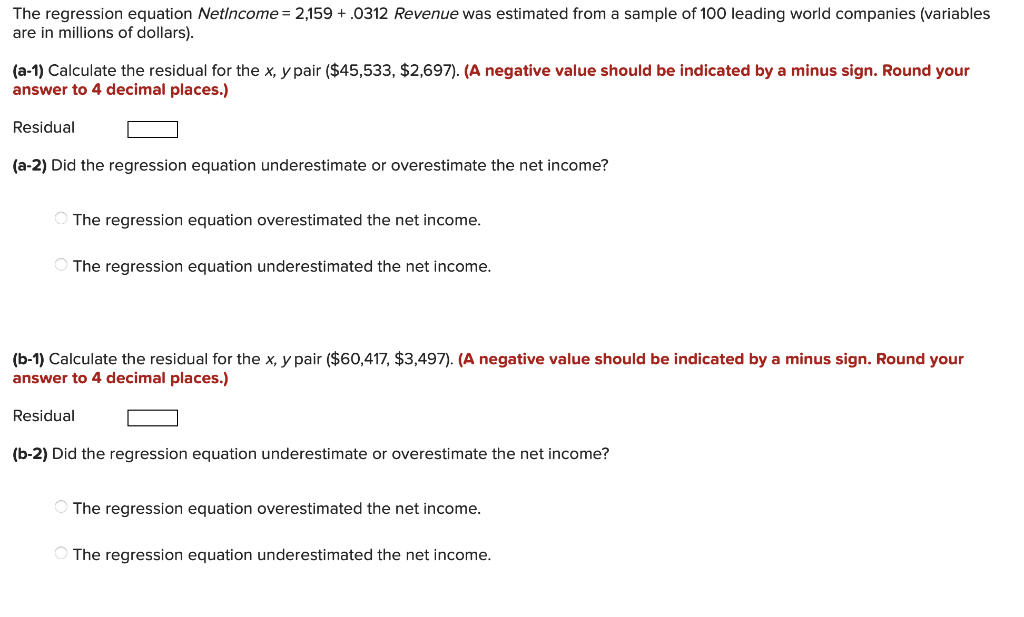# Question The regression equation Netlncome = 2,159 +.0312 Revenue was estimated from a sample of 100 leading world companies (variables are in millions of dollars). (a-1) Calculate the residual for the x, y pair (\$45,533, \$2,697). (A negative value should be indicated by a minus sign. Round your answer to 4 decimal places.) Residual (a-2) Did the regression equation underestimate or overestimate the net income? The regression equation overestimated the net income. The regression equation underestimated the net income. (6-1) Calculate the residual for the x, y pair (\$60,417, \$3,497). (A negative value should be indicated by a minus sign. Round your answer to 4 decimal places.) Residual (b-2) Did the regression equation underestimate or overestimate the net income? The regression equation overestimated the net income. The regression equation underestimated the net income.YXYORU The Asker · Probability and StatisticsTranscribed Image Text: The regression equation Netlncome = 2,159 +.0312 Revenue was estimated from a sample of 100 leading world companies (variables are in millions of dollars). (a-1) Calculate the residual for the x, y pair (\$45,533, \$2,697). (A negative value should be indicated by a minus sign. Round your answer to 4 decimal places.) Residual (a-2) Did the regression equation underestimate or overestimate the net income? The regression equation overestimated the net income. The regression equation underestimated the net income. (6-1) Calculate the residual for the x, y pair (\$60,417, \$3,497). (A negative value should be indicated by a minus sign. Round your answer to 4 decimal places.) Residual (b-2) Did the regression equation underestimate or overestimate the net income? The regression equation overestimated the net income. The regression equation underestimated the net income.
More
Transcribed Image Text: The regression equation Netlncome = 2,159 +.0312 Revenue was estimated from a sample of 100 leading world companies (variables are in millions of dollars). (a-1) Calculate the residual for the x, y pair (\$45,533, \$2,697). (A negative value should be indicated by a minus sign. Round your answer to 4 decimal places.) Residual (a-2) Did the regression equation underestimate or overestimate the net income? The regression equation overestimated the net income. The regression equation underestimated the net income. (6-1) Calculate the residual for the x, y pair (\$60,417, \$3,497). (A negative value should be indicated by a minus sign. Round your answer to 4 decimal places.) Residual (b-2) Did the regression equation underestimate or overestimate the net income? The regression equation overestimated the net income. The regression equation underestimated the net income.